## My 1975 IMO Team

I just got this picture from my friend Victor Gutenmacher, which I never saw before. My 1975 IMO team is posing at our training grounds before the Olympiad trip to Bulgaria.

Left to right: Boris Yusin, Yuri Ionin, Zoya Moiseyeva (front), Gregory Galperin (back), me, Ilya Yunus, Valentin Skvortsov, Aleksandr Kornyushkin, Sergei Finashin, Sergei Fomin (front), Alexander Reznikov (back), Yuri Shmelev (front), Yuri Neretin (back), Victor Gutenmacher.

Our coaches are in the shot as well. Surprisingly, or not surprisingly, all of them moved to the USA. Yuri Ionin, now retired, was a professor at Central Michigan University. Gregory Galperin is a professor at Eastern Illinois University. Sergei Fomin is a professor at the University of Michigan. Victor Gutenmacher worked for BBN Technologies and Siemens PLM Software, and is now retired.

There are two more adults in the picture: Valentin Anatolievich Skvortsov, our leader and Zoya Ivanovna Moiseyeva, our deputy leader. Skvortsov was working at the math department of Moscow State University at that time. The University was angry that he didn’t block some students with Jewish heritage from the team thus allowing them to be accepted to Moscow State University without exams. I wrote a story of how Zoya persuaded Alexander Reznikov not to go to Moscow University to help Valentin. It ruined Alexander’s live, and didn’t even help Valentin. 1975 was Valentin’s last trip as the leader.

Share:

## The Annoyance of Hyperbolic SurfacesI do not like making objects with my hands. But I lived in Soviet Russia. So I knew how to crochet, knit, and sew — because in Russia at that time, we didn’t have a choice. I was always bad at it. The only thing I was good at was darning socks: I had to do it too often. By the way, I failed to find a video on how to darn socks the same way my mom taught me.

Then I came to the US. I suddenly found myself in a rich society, where it was cheaper to buy new stuff than to spend the time doing things with my hands. So I happily dropped my craftsmanship.

After not working with my hands for 28 years, one day I needed hyperbolic surfaces for my classes and I couldn’t find any to buy. Hyperbolic surfaces are famous for providing an example when the Euclid’s Fifth axiom doesn’t work. These hyperbolic surfaces look flat locally, so you can continue a line in any given direction. If you draw a line on such a surface and pick a point that is not on the line, then you can draw many lines through the point that are parallel to the given line.

My students are more important than my dislike of crochet, so I decided to just do it myself. I asked my friend Debbie, who knows how to crochet, for advice, and she gave me more than advice. She gave me a hook and a piece of yarn and reminded me how to work the hook. She started me with a small circle. After that all I had to do was add two stitches for each stitch on the perimeter of the circle. The finished product is this green ballish thing that looks like a brain, as in the photo.

Outside the starting circle, each small surface segment of this “brain” looks the same, making the “brain” a surface of constant curvature.

I chose a ratio of 2 to 1, adding two new stitches for each previous stitch. With this ratio, my flattish surface started looking like a ball very fast. The length of the perimeter doubled for every row. Thus each new row I crocheted took the same total amount of time that I had already spent on the whole thing. All the hours I worked on this “brain,” I kept thinking: darn, it is so unrewarding to do this. Annoying as it was, the thing that kept me going was my initial decision to continue to use up all the yarn Debbie had given me. In the end, with this ratio, half the time I worked was spent making the final row.Share:

## 3-Inflatable Permutations

We can inflate one permutation with another permutation. Let me define the inflation by examples and pictures. Suppose we have a permutation 132 which we want to inflate using permutation 21. The result is the permutation 216543 that can be divided into three blocks 21|65|43. The blocks are ordered as the first permutation 132, and within each block the order is according to the second permutation. This operation is often called a tensor product of two permutations. The operation is non-commutative: the inflation of 21 with 132 is 465132.As one might guess this post is related to k-symmetric permutations, that is, permutations that contain all possible patterns of size k with the same frequency. As I mentioned in my recent post 3-Symmetric Permutations, the smallest non-trivial examples of 3-symmetric permutations are 349852167 and 761258943 in size 9.

A permutation is called k-inflatable if its inflation with k-symmetric permutation is k-symmetric. One of my PRIMES projects was about 3-inflatable permutations. The result of this project is the paper On 3-Inflatable Permutations written with Eric Zhang and posted at the arxiv.

The smallest non-trivial examples of 3-inflatable permutations are in size 17: E534BGA9HC2D1687F and B3CE1H76F5A49D2G8, where capital letters denote numbers greater than nine. Another cool property discovered in the paper is that the tensor product of two k-inflatable permutations is k-inflatable.Share:

## Symmetries of k-Symmetric Permutations

I am fascinated by 3-symmetric permutations, that is, permutations that contain all possible patterns of of size three with the same frequency. As I mentioned in my recent post 3-Symmetric Permutations, the smallest non-trivial examples are in size 9.

When I presented these examples at a combinatorics pre-seminar, Sasha Postnikov suggested to draw the permutations as a graph or a matrix. Why didn’t I think of that?

Below are the drawings of the only two 3-symmetric permutations of size 9: 349852167 and 761258943.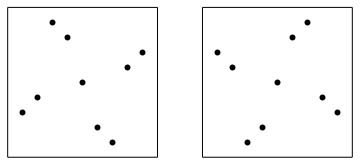As I already mentioned in the aforementioned essay the set of 3-symmetric permutations is invariant under the reversal and subtraction of each number from the size of the permutation plus 1. In geometrical terms it means reflection along the vertical midline and central symmetry. But as you can see the pictures are invariant under 90 degree rotation. Why?

What I forgot to mention was that the set of k-symmetric permutations doesn’t change after the inversion. In geometrical terms it means the reflection with respect to the main diagonal. If you combine a reflection with respect to a diagonal with a reflection with respect to a vertical line you get a 90 degree rotation. Overall, the symmetries of the k-symmetric permutations are the same as all the symmetries of a square. Which means we can only look at the shapes of the k-symmetric permutations.

There are six 2-symmetric permutations: 1432, 2341, 2413, 3142, 3214, 4123. As we can see in the picture below they have two different shapes.Here is the list of all 22 2-symmetric permutations of size 5: 14532, 15342, 15423, 23541, 24351, 24513, 25143, 25314, 31542, 32451, 32514, 34152, 34215, 35124, 41352, 41523, 42153, 42315, 43125, 51243, 51324, 52134. The list was posted by Drake Thomas in the comments to my essay. Up to symmetries the permutations form four groups. Group 1: 14532, 15423, 23541, 32451, 34215, 43125, 51243, 52134. Group 2: 15342, 24351, 42315, 51324. Group 3: 24513, 25143, 31542, 32514, 34152, 35124, 41523, 42153. Group 4: 25314, 41352. The picture shows the first permutation in each group.## 3-Symmetric Graphs

In my previous post I described 3-symmetric permutations. Now I want to define 3-symmetric graphs.

A reminder: a k-symmetric permutation is such that the densities of all permutations of size k in it are the same. In particular a 2-symmetric permutation has the same number of inversions and non-inversions. How do we translate this to graphs? I call a graph 2-symmetric if it has the same number of edges as non-edges. 2-symmetric graphs are easy to construct, but they only exist if the number of vertices, n, is such that n choose 2 is even. The simplest non-trivial examples are graphs with 4 vertices and three edges.

The above definition is difficult to generalize. So I rephrase: a graph G is 2-symmetric, if the density of any subgraph H with 2 vertices in G is the same as the expected density of H in a random graph where the probability of an edge equals 1/2. This definition is easy to generalize. A graph G is k-symmetric, if the density of any subgraph H with k vertices in G is the same as the expected density of H in a random graph where the probability of an edge equals 1/2. In particular, here are the densities of all four possible subgraphs with 3 vertices in a 3-symmetric graph:

• A complete graph with 3 vertices: 1/8,
• A path graph with 3 vertices: 3/8,
• A graph with 3 vertices and only one edge: 3/8,
• A graph with 3 isolated vertices: 1/8.

For a graph G to be 3-symmetric, the number of vertices, n, in G needs to be such that n choose 3 is divisible by 8. The first non-trivial case is n = 8. Here are the pictures of two 3-symmetric graphs. The first one is a wheel, and the second one is its complement.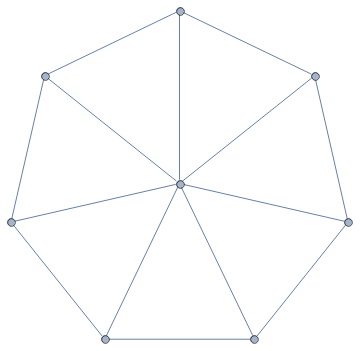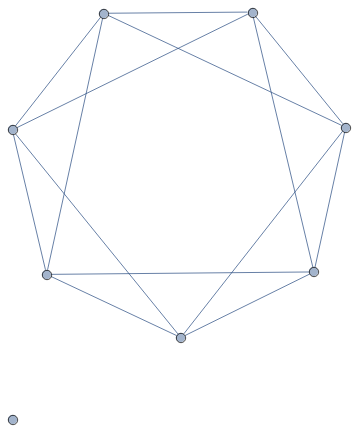## 3-Symmetric Permutations

I want to study patterns inside permutations. Consider a permutation of size 4: 1432. Today I am excited by ordered subset of size 3 inside this permutation. For example, I can drop the last number and look at 143. The ordering in 143 is the same as in 132, or, as a mathematician would say, 143 is order-isomorphic to 132. In my example of 1432, I have four subsets depending on which number I remove: 432 is order-isomorphic to 321, while 132, 142 and 143 are all order-isomorphic to 132.

The density of a pattern 123 in the permutation 1432 is the ratio of subsets of size 3 that are order-isomorphic to 123 to all subsets of size 3. As I already calculated, the density of 123 in 1432 is zero, while the density of 321 is 1/4, and the density of 132 is 3/4.

A permutation is called 3-symmetric if the densities of all patterns of size 3 (123, 132, 213, 231, 312, 321) are the same. The reason I love this notion, is that 3-symmetric permutations are similar to random permutations with respect to patterns of size 3.

My example permutation, 1432, is not 3-symmetric. Thinking about it, no permutation of size 4 can be 3-symmetric. The number of subsets of size 3 is four, which is not divisible by 6.

I wanted to find 3-symmetric permutations. So the size n of the permutation needs to be such that n choose 3 is divisible by 6. The numbers with this property are easy to find. The sequence starts as 1, 2, 9, 10, 18, 20, 28, 29, 36, 37, 38, 45, 46. The sequence is periodic with period 36.

Any permutation of sizes 1 or 2 is 3-symmetric as all densities are zero. Duh!

The next interesting size is 9. My student, Eric Zhang, wrote a program and found that there are two 3-symmetric permutations of size 9: 349852167 and 761258943. These numbers are so cool!. First, they are reverses of each other. This is not very surprising: if a permutations is 3-symmetric, then its reverse must also be 3-symmetric. There is another property: the permutations are rotational symmetries of each other. That is, the sum of two digits in the same place is 10. You can see that rotating a 3-symmetric permutation produces a 3-symmetric permutation.

I decided to write a program to find 3-symmetric permutations of the next size: 10. There is none. I do not trust my programming skills completely, so adjusted my program to size 9 and got the same result as Eric. I trust Eric’s programming skills, so I am pretty sure that there are no 3-symmetric permutations of size 10. Maybe there are some 3-symmetric permutations in size 18.

Let’s find 2-symmetric permutations. These are permutations with the same number of ascends and descends inversions and non-inversions. For n to be the size of such permutation n choose 2 needs to be divisible by 2. That means n has to have remainder 0 or 1 modulo 4. The first nontrivial case is n = 4. There are six 2-symmetric permutations: 1432, 2341, 2413, 3142, 3214, 4123. We can also group them into reversible pairs: 1432 and 2341, 2413 and 3142, 3214 and 4123. If we look at rotational symmetry we get different pairs: 1432 and 4123, 2341 and 3214, 2413 and 3142.

You can try to find non-trivial 4-symmetric permutations. Good luck! The smallest nontrivial size is 33. Finding 5-symmetric permutations is way easier: the smallest nontrivial size is 28. The sequence of nontrivial sizes as a function of n is: 1, 4, 9, 33, 28, 165, 54, 1029, 40832, 31752, 28680, 2588680, 2162700, and so on. My computer crashed while calculating it.Share:

## Mafia Logic Puzzle

I found this puzzle on the Russian QWERTY channel.

Five people sit around a table playing Mafia. Among them are two innocent people, two Mafiosos, and one detective. The Mafia people know each other; the detective knows who each of them is; and the innocent people have no information whatsoever about anyone at the table.

During this particular game, the innocents and the detective always tell the truth, while mafia people always lie. They start by going around the circle making the following statements:

• A: I know who B is.
• B: I know who the detective is.
• C: I know who B is.
• D: I know who E is.

Who is who?

## Shapes of Symbols in Latin Squares

Once John Conway showed me a cute way to enumerate Latin squares of size 4, up to the movements of the plane. It was a joint result with Alex Ryba, which is now written in a paper Kenning KenKen.

For starters, I want to remind you that a Latin square of size n is an n by n table filled with integers 1 through n, so that every row and column doesn’t have repeated integers. KenKen is a game that John Conway likes, where you need to recover a Latin square given some information about it.

Let me start by describing a particular shape of four cells that one digit can occupy in a Latin square of size 4. There are only seven different shapes. To get to the beautiful result, we need to number these seven shapes in a particular order starting from zero. The shapes are shown below.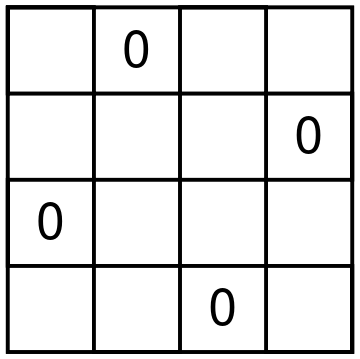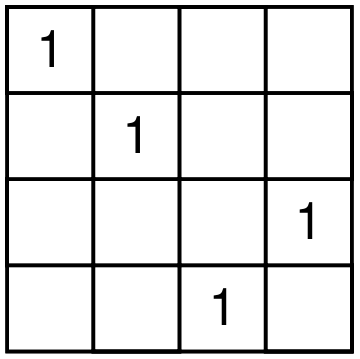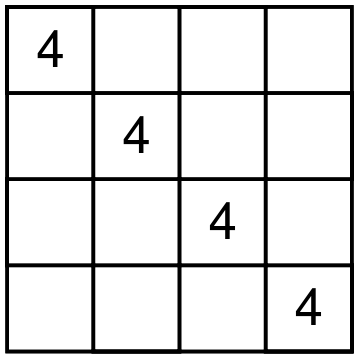There are 12 different Latin squares up to movements of the square and relabeling the digits. Here is how Conway and Ryba matches shapes and squares. For each Latin square, take the shapes of all four digits, remove the duplicate shape numbers and sum the leftover shape numbers. You will get a unique number from 1 to 12 that represents a particular Latin square. For example, consider the square on the picture below.Digit 1 is shape 4, digits 2 and 4 form shape 2, and digit 3 forms shape 6. Shape 2 is used twice, and we ignore multiplicities. So we have shapes 2, 4, and 6 used. The resulting Latin square is number 2 + 4 + 6, that is 12. It is a fun exercise to try to find all the squares. For example, square 1 can only use shapes 0 and 1. But shape 1 uses exactly one corner. So the first square should use each of the digits in shape 1.

John likes finding interesting ways to remember which shape is which. You can find his and Alex’s suggestions in the paper which Alex submitted to the arxiv.

## Another Cool Coin-Weighing Problem

My coauthor, Konstantin Knop, sent me a coin-weighing problem that is really good. Surprisingly, it is old: it first appeared in a Russian math journal, Kvant, in 1973.

Puzzle. At a trial, 14 coins were presented as material evidence. The expert tested the coins and discovered that seven of them were fake, the rest were real, and he knew exactly which coins were fake and which were real. The court only knows that counterfeit coins weigh the same, real coins weigh the same, and fake ones are lighter than real ones. The expert wants to use not more than three weighings on a balance scales without weights to prove to the court that all the counterfeit coins he found are really fake, and the rest are real. Could he do it?